## RD Sharma Class 10 Solutions Chapter 6 Co-ordinate Geometry VSAQS

These Solutions are part of RD Sharma Class 10 Solutions. Here we have given RD Sharma Class 10 Solutions Chapter 6 Co-ordinate Geometry VSAQS

Other Exercises

Answer each of the following questions either in one word or one sentence or as per requirement of the questions :
Question 1.
Write the distance between the points A (10 cosθ, 0) and B (0, 10 sinθ).
Solution:
Distance between the points A (10 cosθ, 0) and B (0, 10 sinθ)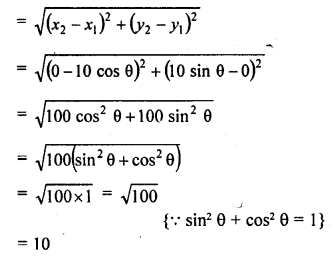Question 2.
Write the perimeter of the triangle formed by the points O (0, 0), A (a, 0), and B (0, b).
Solution:
The vertices of a ∆OAB, O (0, 0), A (a, 0), and B (0, b)
Now length of OA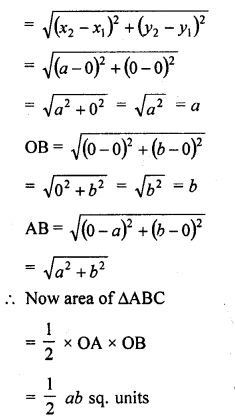Question 3.
Write the ratio in which the line segment joining points (2, 3) and (3, -2) is divided by x-axis.
Solution:
The required point is on x-axis
Its ordinate will be 0
Let the point be (x, 0) and let this point divides the join of the points (2, 3) and (3, -2) in the ratio m : n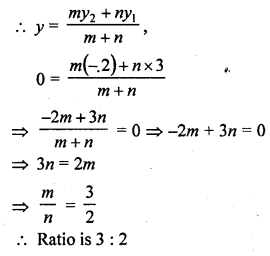Question 4.
What is the distance between the points (5 sin 60°, 0) and (0, 5 sin 30°) ?
Solution:
Distance between the given pointsQuestion 5.
If A (-1, 3), B (1, -1) and C (5, 1) are the vertices of a triangle ABC, what is the length of the median through vertex A ?
Solution:
The vertices of ∆ABC are A (-1, 3), B (1, -1) and C (5, 1)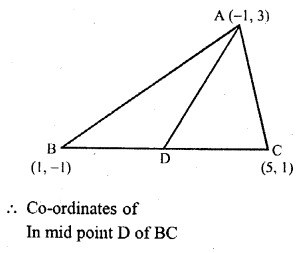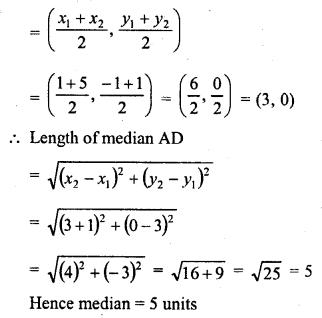Question 6.
If the distance between points (x, 0) and (0, 3) is 5, what are the value of x ?
Solution:
Distance between (x, 0) and (0, 3) = 5Question 7.
What is the area of the triangle formed by the points O (0, 0), A (6, 0) and B (0, 4) ?
Solution:
The vertices of the triangle OAB are O (0, 0), A (6, 0) and B (0, 4)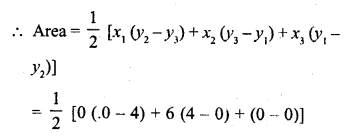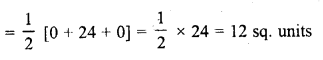Question 8.
Write the coordinates of the point dividing line segment joining points (2, 3) and (3, 4) internally in the ratio 1 : 5.
Solution:
Let the coordinates of the required point be (x, y), thenQuestion 9.
If the centroid of the triangle formed by points P (a, b), Q (b, c) and R (c, a) is at the origin, what is the value of a + b + c ?
Solution:
Vertices of ∆PQR are P (a, b), Q (b, c) and R (c, a) and its centroid = O (0, 0)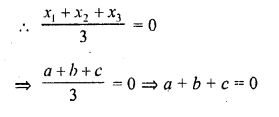Question 10.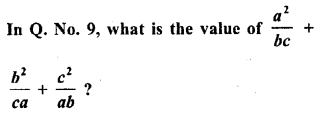Solution: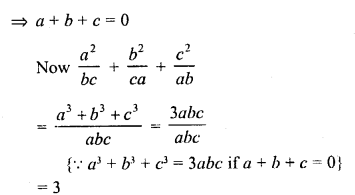Question 11.
Write the coordinates of a point on x- axis which is equidistant from the points (-3, 4) and (2, 5).
Solution:
The point is on x-axis
Its ordinates of the point P is (x, 0)
P is equidistant from A (-3, 4) and B (2, 5)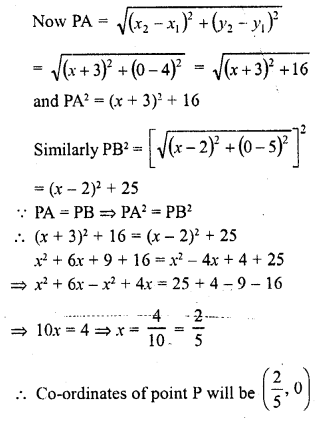Question 12.
If the mid-point of the segment joining A (x, y + 1) and B (x + 1, y + 2) is C ($$\frac { 3 }{ 2 }$$ , $$\frac { 5 }{ 2 }$$) find x, y.
Solution:
C ($$\frac { 3 }{ 2 }$$ , $$\frac { 5 }{ 2 }$$) is mid point of the line segment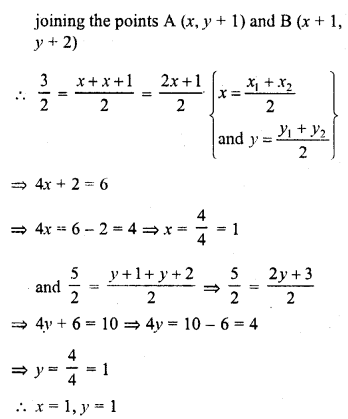Question 13.
Two vertices of a triangle have co-ordinates (-8, 7) and (9, 4). If the centroid of the triangle is at the origin, what are the co-ordinates of the third vertex ?
Solution:
Two vertices of a triangle are (-8, 7) and (9, 4)
Let the third vertex be (x, y)
Centroid of the triangle is (0, 0)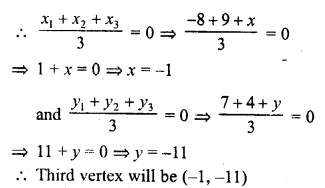Question 14.
Write the coordinates the reflections of points (3, 5) in x and y-axes.
Solution:
Reflection of P (3, 5) in x-axis is will be (3, -5)
and reflection of P in y-axis will be (-3, 5)

Question 15.
If points Q and R reflections of point P (-3, 4) in X and Y axes respectively, what is QR ?
Solution:
Reflection of point P (-3, 4) in X-axis will be Q with coordinates Q (-3, -4) and reflection in Y-axis will be R (3, 4)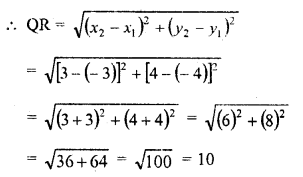Question 16.
Write the formula for the area of the triangle having its vertices at (x1, y1), (x2, y2) and (x3, y3).
Solution:Question 17.
Write the condition of collinearity of points (x1, y1), (x2, y2) and (x3, y3).
Solution:
Three points (x1, y1), (x2, y2) and (x3, y3). are said to be collinear if the area of the triangle formed by these point = 0 i.e.,Question 18.
Find the values of x for which the distance between the point P (2, -3) and Q (x, 5) is 10.
Solution:
Distance between P (2, -3) and Q (x, 5) = 10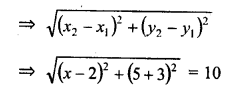Question 19.
Write the ratio in which the line segment joining the points A (3, -6) and B (5, 3) is divided by X-axis.
Solution:
The point lies on x-axis
Its ordinate will be = 0
Let the point P (x, 0) divides the line segment joining the points A (3, -6) and B (5, 3) in the ratio m : n.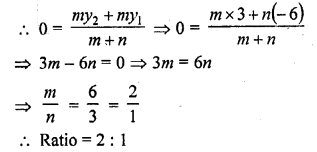Question 20.
Find the distance between the points ($$\frac { -8 }{ 5 }$$ , 2) and ($$\frac { 2 }{ 5 }$$ , 2). (C.B.S.E. 2009)
Solution:Question 21.
Find the value of a so that the point (3, a) lies on the line represented by 2x – 3y + 5 = 0. (C.B.S.E. 2009)
Solution:Question 22.
What is the distance between the points A (c, 0) and B (0, – c) ? [CBSE 2010]
Solution: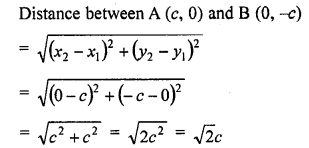Question 23.
If P (2, 6) is the mid-point of the line segment joining A (6, 5) and B (4, y), find y. [CBSE 2010]
Solution:
P (2, 6) is the mid-point of the line segment A (6, 5) and b (4, y)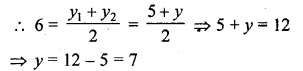Question 24.
If the distance between the points (3, 0) and (0, y) is 5 units and y is positive, then what is the value of y ? [CBSE 2010]
Solution:
Distance between (3, 0) and (0, y) is 5 unitsQuestion 25.
If P (x, 6) is the mid-point of the line segment joining A (6, 5) and B (4, y), find y. [CBSE 2010]
Solution:
P (x, 6) is the mid-point of the line segment joining the points A (6, 5), B (4, y)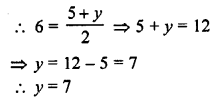Question 26.
If P (2, p) is the mid-point of the line segment joining the points A (6, -5) and B (-2, 11), find the value of p. [CBSE 2010]
Solution:
P (2, p) is the mid-point of the line segment joining the points A (6, -5) and B (-2, 11)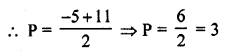Question 27.
If A (1, 2), B (4, 3) and C (6, 6) are the three vertices of a parallelogram ABCD, find the coordinates of fourth vertex D. [CBSE 2010]
Solution:
vertices of a parallelogram Let co-ordinates of D be (x, y)
Diagonals AC and BD bisect each other at O
Co-ordinates of O will be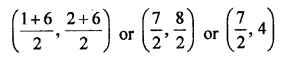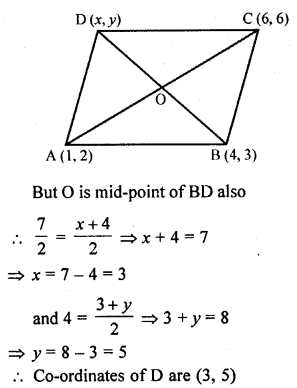Question 28.
What is the distance between the points A (sinθ – cosθ, 0) and B (0, sinθ + cosθ)? [CBSE 2015]
Solution:Question 29.
What are the coordinates of the point where the perpendicular bisector of the line segment joining the points A (1, 5) and B (4, 6) cuts the y-axis?
Solution:
Firstly, we plot the points of the line segment on the paper and join them.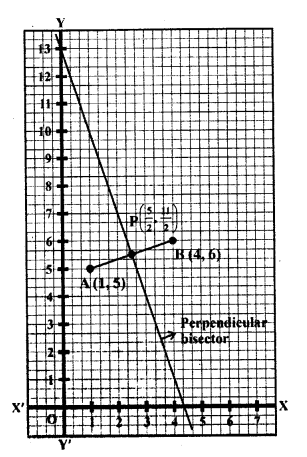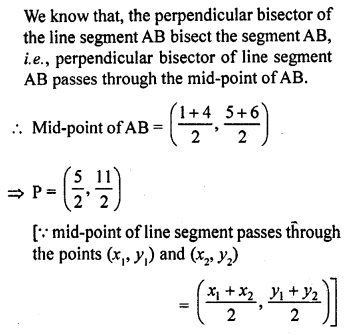Now, we draw a straight line on paper passes through the mid-point P.
We see that the perpendicular bisector cuts the y-axis at the point (0, 13).
Hence, the required point is (0, 13).

Question 30.
Find the area of the triangle with vertices (a, b + c), (b, c + a) and (c, a + b).
Solution: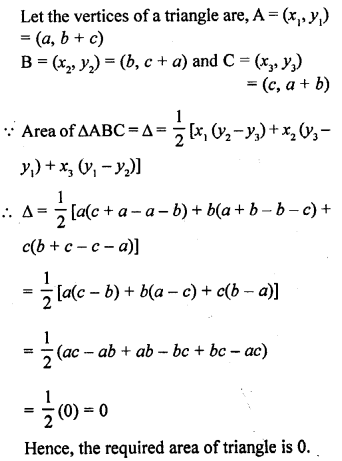Question 31.
If the points A (1, 2), O (0, 0) and C (a, b) are collinear, then find a : b.
Solution: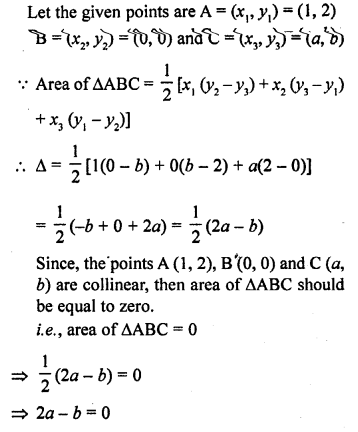=> 2a = b
Hence, the required relation is 2a = b

Question 32.
Find the coordinates of the point which is equidistant from the three vertices A (2x, 0), O (0, 0) and B (0, 2y) of ∆AOB.
Solution:
Let the coordinate of the point which is equidistant from the three vertices O (0, 0), A (0, 2y) and B (2x, 0) is P (h, k).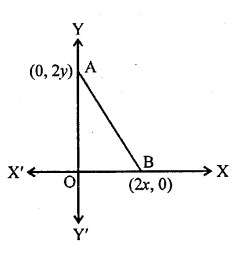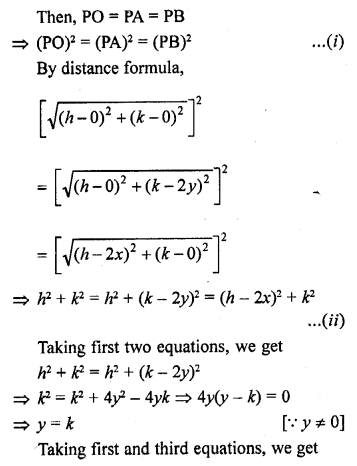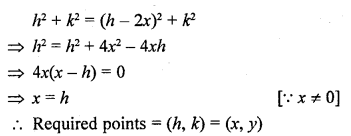Question 33.
If the distance between the points (4, k), and (1, 0) is 5, then what can be the possible value of k? [CBSE 2017]
Solution:
Let the points x (4, k) and y (1, 0)
It is given that the distance xy is 5 units.
By using the distance formula,Hope given RD Sharma Class 10 Solutions Chapter 6 Co-ordinate Geometry VSAQS are helpful to complete your math homework.

If you have any doubts, please comment below. Learn Insta try to provide online math tutoring for you.